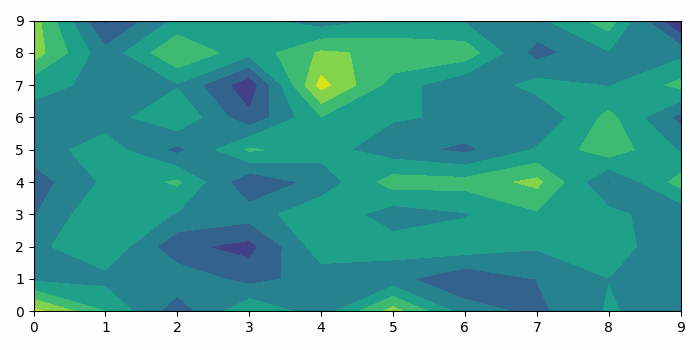# How to use matplotlib.animate to animate a contour plot in Python?

To animate a contour plot in matplotlib in Python, we can take the following steps−

• Create a random data of shape 10☓10 dimension.
• Create a figure and a set of subplots using subplots() method.
• Makes an animation by repeatedly calling a function *func* using FuncAnimation() class.
• To update the contour value in a function, we can define a method animate that can be used in FuncAnimation() class.
• To display the figure, use show() method.

## Example

import numpy as np
import matplotlib.pyplot as plt
import matplotlib.animation as animation
plt.rcParams["figure.figsize"] = [7.00, 3.50]
plt.rcParams["figure.autolayout"] = True
data = np.random.randn(800).reshape(10, 10, 8)
fig, ax = plt.subplots()
def animate(i):
ax.clear()
ax.contourf(data[:, :, i])
ani = animation.FuncAnimation(fig, animate, 5, interval=50, blit=False)
plt.show()

## Output